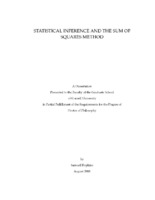## Statistical Inference and the Sum of Squares Method##### Abstract
Statistical inference on high-dimensional and noisy data is a central concern of modern computer science. Often, the main challenges are inherently computational: the problems are well understood from a purely statistical perspective, but key statistical primitives -- likelihood ratios, Bayes-optimal estimators, etc. -- are intractable to compute on large and high-dimensional data sets. We develop a unified approach to algorithm design for statistical inference based on the Sum of Squares method, a powerful tool for convex programming with low-degree polynomials, which generalizes linear programming and spectral algorithms. We use this approach to design algorithms for a range of high-dimensional statistical problems, improving on state-of-the-art provable guarantees in several well-studied settings: clustering data from high-dimensional mixture models, community detection in random graphs, and more. We also prove computational lower bounds for some statistical problems, including the long-studied planted clique problem. Our lower bounds provide new strong evidence for the existence of information-computation gaps -- that is, statistical problems which are solvable given infinite computational resources, but not by efficient algorithms. In particular, we prove new lower bounds against the powerful Sum of Squares hierarchy of semidefinite programs, via a new technique called "pseudocalibration". Because the Sum of Squares hierarchy has provable guarantees matching those of most known techniques in algorithm design for inference, our lower bounds strongly suggest that the problems we study are intractable for polynomial-time algorithms. At very least, improving existing algorithms for them would require a major breakthrough. We show that polynomial-size semidefinite programs from the Sum of Squares hierarchy cannot refute the existence of cliques of size much less than the square root of n in n-node random graphs. Additionally, we prove a lower bound for sparse principal component analysis (PCA), showing that subexponential-size Sum of Squares semidefinite programs are needed to improve on the provable guarantees of existing spectral algorithms for sparse PCA. Our approach to algorithms and lower bounds suggests a new method to chart the edge of algorithmic tractability for statistical inference. We propose a classification of Bayesian inference problems according to solvability by algorithms which compute only simple statistics of input data -- triangle counts of graphs, top eigenvalues of matrices, etc. Our classification accurately predicts suspected information-computation gaps for many well-studied problems, including planted clique, planted constraint satisfaction, community detection in stochastic block models, component analysis problems, and more. This type of classification is novel for inference problems, and represents the first approach to trace the information-computation gaps in of all these problems to the same underlying mathematical structure.
2018-08-30
##### Keywords
Statistics; Computational Complexity; Semidefinite Programming; Algorithms; Sum of Squares Method; Mathematics; Computer science; Spectral Methods; Statistical Inference
Steurer, David
##### Committee Member
Kozen, Dexter Campbell
Kleinberg, Robert David
Computer Science
##### Degree Name
Ph. D., Computer Science
##### Degree Level
Doctor of Philosophy